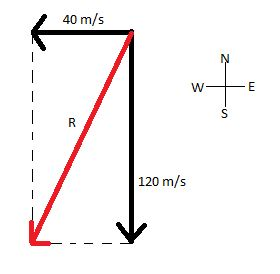# The pilot of a plane points his airplane due South and flies with an airspeed of 120 m/s....

## Question:

The pilot of a plane points his airplane due South and flies with an airspeed of 120 m/s. Simultaneously, there is a steady wind blowing due West with a constant speed of 40 m/s.

a. Make a sketch that shows how to find the resultant velocity of the plane. Roughly in what direction is the resultant velocity?

b. What is the resultant speed of the airplane?

c. After one hour, how far away is the plane from its starting point?

This problem involves sketching the vector diagram of the given scenario using appropriate vectors. Then we need to find the resultant speed of the airplane using simple vector addition. Later, we need to find the location of the plane from the starting point, which we calculated using the formula, distance is equal to speed times time taken.

Given the pilot of a plane points his airplane due south and flies with an airspeed of 120 m/s. There is a steady wind blowing due west with a constant speed of 40 m/s.

(a) Sketch by which we can find the resultant velocity(b) The resultant speed of the airplane is the vector addition of the two vectors in perpendicular addition. So we have,

{eq}\displaystyle R = \sqrt { 120^2 + 40^2 } = 126.4911 {/eq} m/s

(c) Location of the plane one hour after leaving its starting point.

We can find the distance it traveled in the south direction and west direction, thus find the exact location.

{eq}\displaystyle d_{south} = 120 * 60 * 60 = 432 {/eq} km

{eq}\displaystyle d_{west} = 40* 60*60 = 144 {/eq} km

So, the location is 432 km south and 144 km west of the starting point. The total distance is {eq}\displaystyle \sqrt { 432^2 + 144^2 } = 455.37 {/eq} km